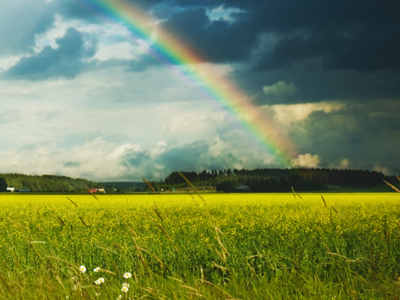The name of a part of the circumference of a circle is arc, such as the arc of a rainbow.

# Shapes (Year 6)

In KS2 Maths, Year Six kids explore cool shapes! Think of a parallelogram, a kite, or a rhombus - they're like shape superheroes! You'll also meet the gang of regular polygons, from circles to dodecagons, and the 3D shape squad. Learn words like circumference, concentric, arc, and parallel – they're the shape secret codes!

Shapes with equal angles, like squares, are called regular. But what about shapes with different angles, like kites? We call them irregular – they have unique names like trapezium or even diamond! Make 3D shapes with card; they appear like magic!

Do you know the difference between a rhombus and a parallelogram? Test your shape skills in this super fun quiz!

1.
How many faces does a dodecahedron have?
8
10
12
14
A regular dodecahedron has pentagon shaped faces
2.
What is the name given to the distance around the edge of circle?
Diameter
Tangent
Circumference
'Circum' means going around and is related to the word 'circle'
3.
Which quadrilateral has no parallel sides?
Square
Kite
Rhombus
Rectangle
A kite has 2 pairs of equal length sides but none of them are parallel to another
4.
Which of these shapes is not a parallelogram?
Square
Rectangle
Rhombus
Trapezium
A parallelogram has all opposite sides parallel and equal in length
5.
What do two concentric circles share?
Centre point
Equal circumference
Equal diameter
Ripples on the surface of a pond are concentric circles
6.
What are perpendicular lines?
Perpendicular lines are lines that are getting closer and closer to each other
Perpendicular lines are lines that are always the same distance apart
Perpendicular lines are lines that are at right angles to each other
Perpendicular lines are lines that are short
The corners of rectangles are formed by perpendicular lines
7.
What is the name of two lines that are always the same distance apart?
Parallel
Perpendicular
Convergent
They never cross each other
8.
A rhombus looks like which other shape?
A kite
A square
A triangle
A diamond
A rhombus is a quadrilateral with 4 equal length sides, like a square that has been squashed!
9.
What is the name of a part of the circumference of a circle?
Arc
Centre
Diameter
Rainbows are arcs
10.
What is the name of a 12 sided flat shape?
Dodecagon
Nonagon
Heptagon
Decagon
'Do' means 2 and 'deca' means 10 so 'dodeca' means 12
You can find more about this topic by visiting BBC Bitesize - Recognise and describe 3D shapes

Author:  Amanda Swift